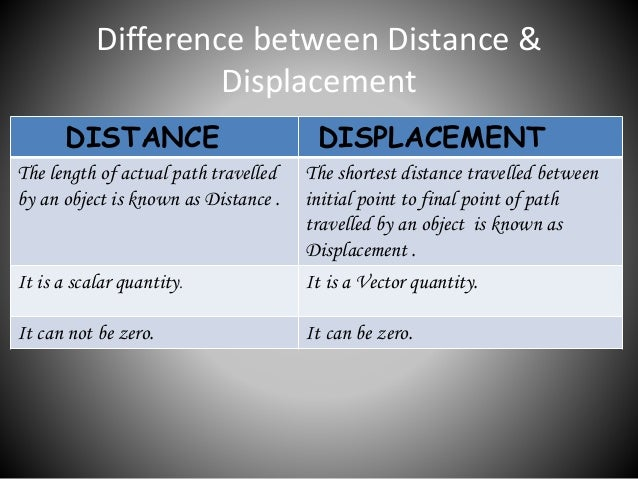# Motion Basics for Class 9th Physics

`The Course Designed based on the pattern of NCERT.Useful and advanced content are added as per Edusaint's Learning framework to enable the student to prepare for IIT foundation and other Relevant exams.`• A tree is fixed at a place and is called stationary
• Similarly, a house, school factory, and poles remain stationary at a place
• However man, animals birds cars buses don’t remain stationary at a place but they can travel from one point to other

#### What Is Motion ?

• We describe the location of an object by specifying a reference point.
• Let us understand this by an example.
• Let us assume that a school in a village is 2 km north of the railway station.
• We have specified the position of the school with respect to the railway station.
• In this example, the railway station is the reference point.
• We could have also chosen other reference points according to our convenience.
• Therefore, to describe the position of an object we need to specify a reference point called the origin.

A body is said to be in motion (or moving) when its position changes continuously with respect to a stationary object taken as a reference point.

For example, a car is moving or it is in motion with respect to stationary objects like house tree,s etc.• In the Above figure the car is at position A in front of the house and a tree at a particular time.
• Here The school and the tree is the reference point
• Now after 30 seconds the car reaches point B which is quite far away from point A. This means the car is changing its position continuously point A to point B with respect to the School and the tree.

Motion can be like playing in a Jhulla, motion can be like moving of the hour clock in a clock or like running like a batsman on the crease.

#### What is Distance traveled and displacement ?

In order to study the meaning of two terms, we need to understand what is distance and displacement

Distance traveled and displacement

• in everyday  world the distance and displace meant are same but in physics both are different• Suppose you want to travel from point A to Point B you can travel on a straight line or also can travel Via C .
• But you traveled via C on the curved path.
• So the distance covered is the (actual distance) you traveled and displacement means the the straight path between the point A and B.

Distance and displacement are two quantities that may seem to mean the same thing yet have distinctly different definitions and meanings.

Distance is a scalar quantity that refers to “how much ground an object has covered” during its motion.
Displacement is a vector quantity that refers to “how far out of place an object is”; it is the object’s overall change in position.

To test your understanding of this distinction, consider the motion depicted in the diagram below.

Your Best friend Shivanee walks 4 meters East, 2 meters South, 4 meters West, and finally 2 meters North.Even though Shivanee has walked a total distance of 12 meters, her displacement is 0 meters. During the course of her motion, she has “covered 12 meters of the ground” (distance = 12 m).
Yet when she is finished walking, she is not “out of place” – i.e., there is no displacement for her motion (displacement = 0 m).

Displacement, being a vector quantity, must give attention to direction.

The 4 meters east cancels the 4 meters west; and the 2 meters south cancels the 2 meters north.

Vector quantities such as displacement are direction aware. Scalar quantities such as distance are ignorant of direction. In determining the overall distance traveled by your friend Shivanee , the various directions of motion can be ignored.

#### A sample Problem for you

What is the displacement of the cross-country team if they begin at the school, run 10 miles and finish back at the school?

Ans. The displacement of the runners is 0 miles. While they have covered a distance of 10 miles, they are not “out of place” or displaced. They finish where they started. Round-trip motions always have a displacement of 0.### MOTION ALONG A STRAIGHT LINE

• The movement of any object from one position to another position with respect to the observer is called Motion. Motion Along a Straight Line:
• When an object moves along a straight line, the motion of the object is called rectilinear motion. For example; the motion of a car on the highway.Scroll to Top